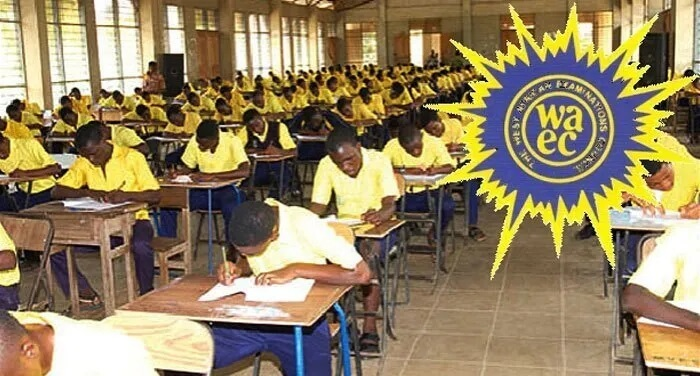# WASSCE 2023: Final Projected Chemistry Questions## These are our final 2023 WASSCE Chemistry projected questions. In this context, you will find the final projected Chemistry questions for the 2023 WASSCE. These Chemistry questions are well analysed to help all WASSCE candidates.

These questions are well analysed from the confirmed topics for Chemistry. Candidates are to note that these are projected questions and not the actual questions they will be writing.

Most of the General Science WASSCE candidates will write their last paper today, 22nd September 2023. Also, most of the General Arts final year students are done with all their papers.

### What Time Is The Elective Chemistry?

The 2023 WASSCE Chemistry paper will be written today, 22nd September 2023. Comparing to the 2022 WASSCE, Chemistry was written on the 23rd September 2022.

Both the Chemistry papers will last for three hours. The Chemistry paper will be in two parts (Section B and Section A). The Subjective part (Section B) will be written for two straight hours (1pm to 3pm) and the Objective part (Section A) will continue right after the paper 2 for an hour (3pm to 4pm).

In all, a total of three hours will be spent on the Chemistry paper.

### Will The 2023 WASSCE Chemistry Paper Be Difficult?

Honestly, Chemistry is one of the easiest subjects to score an A in the WASSCE. With enough effort, a candidate can get at least a B3 in the Chemistry.

The Chemistry paper for the 2023 WASSCE won’t be difficult and easy at the same time, but what is assured is, questions will be set only from the syllabus provided.

### Will The Chemistry Practicals Be Added To The Theory?

Yes, it will. Candidates are to note that, with the Chemistry practicals, there is no rounding up like how the theory will be done.

The total marks for the Chemistry practical are 50 and that of the theory is 140. Only the theory (140 marks) will be converted to 50 marks. So, in order to secure a better grade in Chemistry, you need to do very well in the practical and a little push from the theory as well.

### WASSCE 2023: Final Projected Chemistry Questions

Below, you will find the final projected questions for the Chemistry paper.

1. Define ‘Hydrogen bond’

2. Explain the term ‘dipole-dipole interactions’

3. State Dalton’s law of partial pressure

4. Three gases, 1,2, and 3 has moles a, b, and c respectively. If the total pressure is Pt, then, find the partial pressure of 1,2, and 3.

5. Define a physical property of a substance.

6. List 3 physical properties of concentrated HCl(aq)

7. An ideal gas has the following physical properties: Volume (V), Pressure (P), absolute temperature (T) and the moles (n). Write expressions for the variation of V with :

(i) P at constant T and a fixed n;

(ii) T at constant P and fixed n;

(iii) n at constant T and P.

(iv) Combine all the expressions in (i), (ii) and (iii) to obtain the expression: PV = nRT

8. State, Pauli’s Exclusion Principle.

9. Give one reason why it is not advisable to collect ammonia gas by downward delivery.

10. Define acid-base indicators

11. How does a catalyst affect a reaction at dynamic equilibrium?

12. State Hess’s law of constant heat summation.

13. Using both the collision and activation energy theories, explain how temperature affects the rate of chemical reactions.

READ ALSO: 5 Major Explorers Who Influenced The World With Their Discoveries